The third example extends our approach onto the simulation of gratings. We have the identical S-data tool under the product called OptiGrating, and OptiSystem accepts the data in the same way as in case of the OptiBPM (historically, the grating export/import date were done prior to BPM). This software can characterize miscellaneous optical gratings.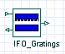The OptiGrating component in the system software is shown on the left. There is just one new attribute having a natural origin. The grating yields two responses from the input signal (the upper left port), the transmission (the right port) and the reflection (the lower-left port).

Let us exploit the assumed “add/drop” circuit at the wavelength 1550 nm. The grating can be generally specified by a few basic parameters, which is shown in Figure 18.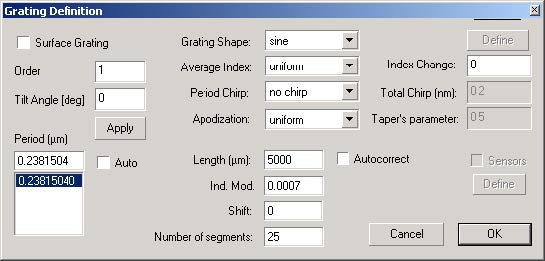Figure 18: Grating parameters dialog box

Here, we deal with basic linear grating, i.e. no period chirp and no apodization are present for the sake of simplicity. Running the calculation produces transmitting and reflecting spectra of the assumed Bragg grating (see Figure 19). These are exported for further use into the OptiSystem. See User Guide of OptiGrating for detailed explanation of the fields.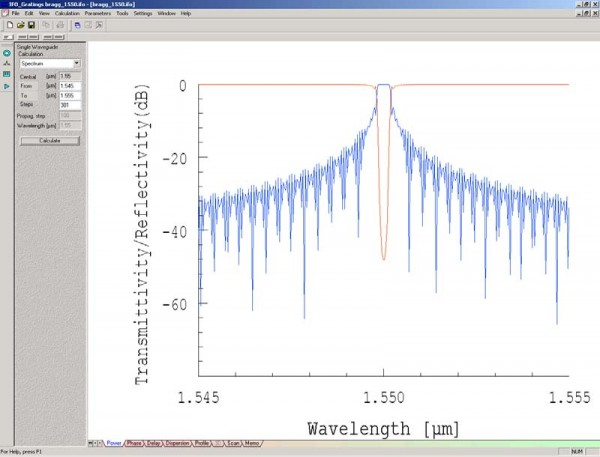Figure 19: Transmission/Reflection calculation results

The add-drop device can be schematically described in OptiSystem as detailed later. However, we must introduce a slight modification of the device design, due to the two output nature of the component. Contrary to the unidirectional BPM component, this bi-directional component possesses one input port and two output ports. Figure 20 shows the schematic representation of the device, where we used the 3dB coupler characterized by the BPM, similar to the previous two examples.
The input coupler plays two roles in the design under study. The former role is the division of the input signal into the identical portions (ideal division into half the power in each arm). The latter one is the recombination of the reflected light from the grating, that happens in the opposite direction to the first one. However, the BPM component cannot accept any kind of bi-directional. We offer the following solution. We simply split the input coupler with the respect to its functionality in both directions as can be seen from the schematics.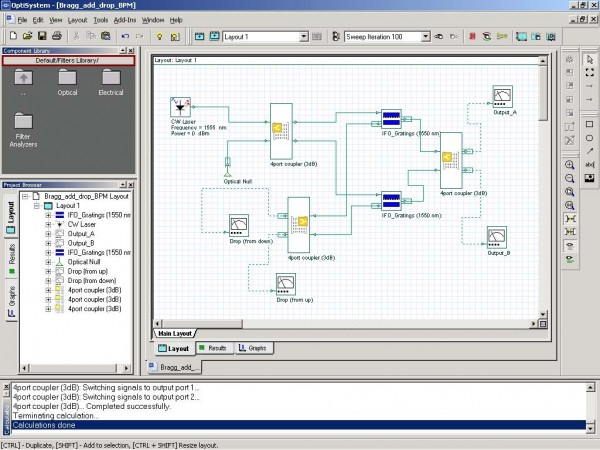Figure 20: Split coupler functionality schematic

The upper input coupler serves as the actual input one (light propagates from left to right – ordinary direction), while the lower coupler acts like that part of the input coupler recombining the reflected signal (backward propagation direction).

The input 3dB coupler is then used three times, but simulated only once by the BPM. The final results from the OptiSystem simulation are shown below (added and dropped signals as the wavelength dependencies. Left – the reflected (dropped) signal with respect to lambda. Right – transmitted spectrum (output B).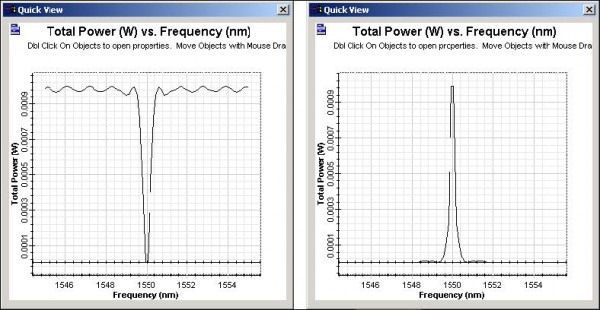Figure 21: Power vs. Frequency calculation results. Added/dropped signals.